# Secant Method Example: Solution for Equation

Find the solution of the following equation using the secant method: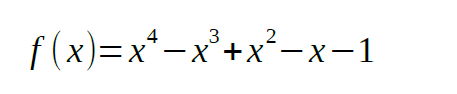Write a Python 3 code for this method.

Solution

Hand solution

Let’s choose the initial values of x: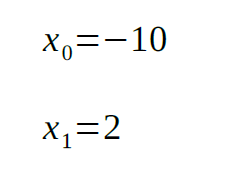Plugging the above values into the equation, we get: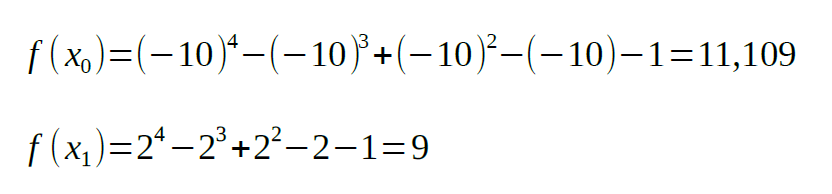The formula for finding the next x values is: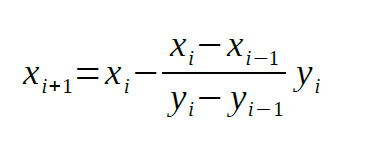Now, we can find the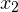value: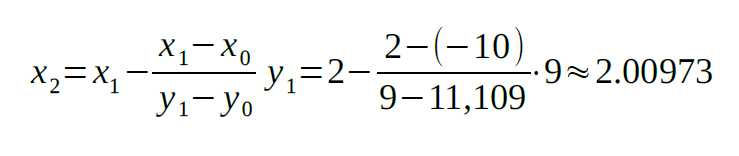Changing x for thevalue: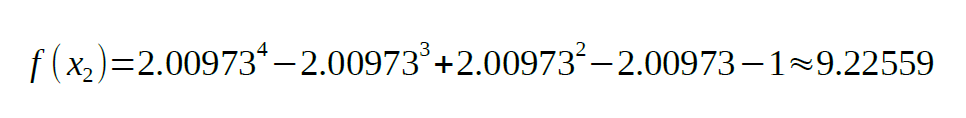Repeating the above steps, we will find the next x values: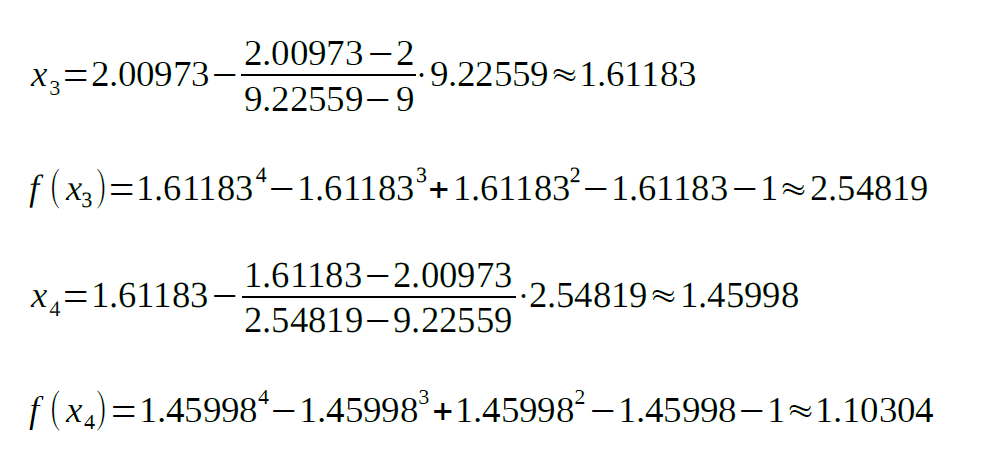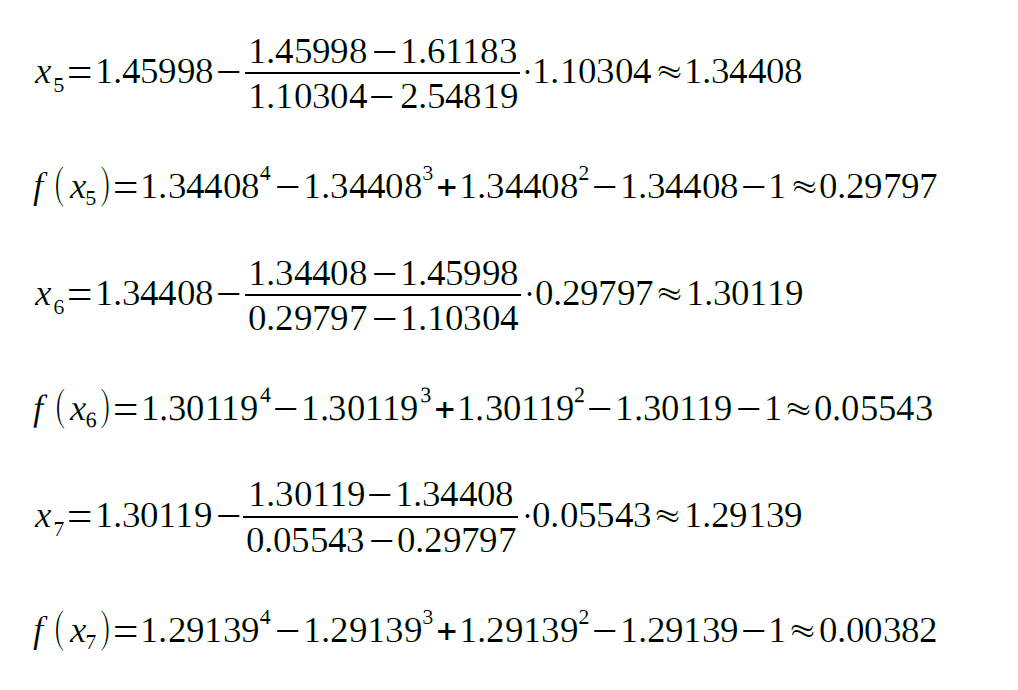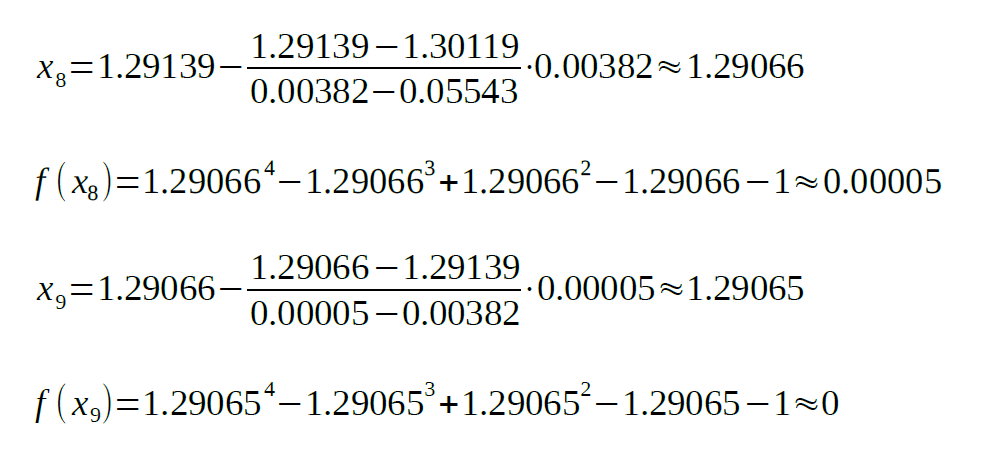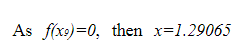is the root of the equation.

Python 3 Code

Let’s define a function for our equation first:Knowing the formula for finding the next values of x, we can write a function for it too: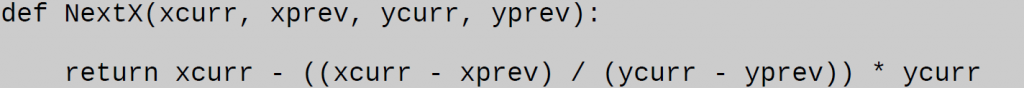Now, it’s time to write our main function for finding the root of the equation. As we should choose two initial values for x, our function should accept two initial parameters: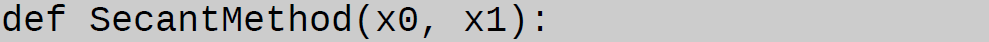Now, let’s find the corresponding y values:To simplify things, we will use current and previous values (xc, yc, xp, yp):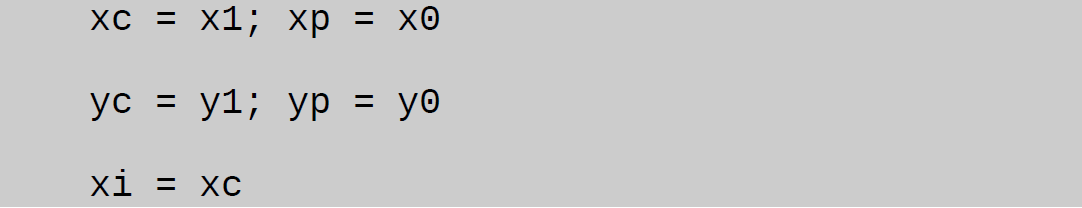And let’s repeat the above steps in a while loop. In order to prevent an infinite loop, we need to add an exit condition. In our case we will check if the absolute value of y is larger than the 0.001 threshold: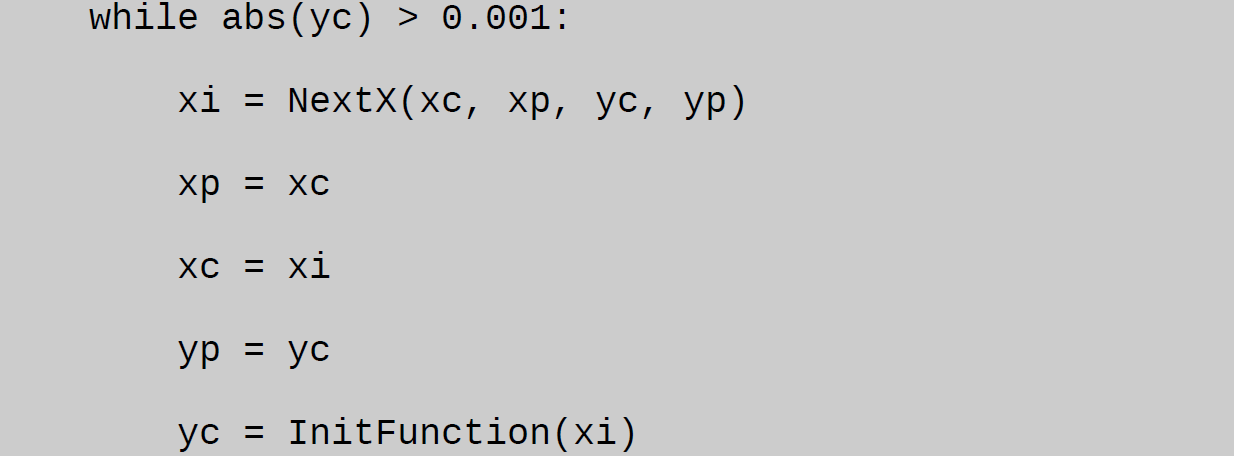At last, the found x value is returned: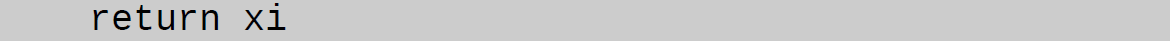The entire code: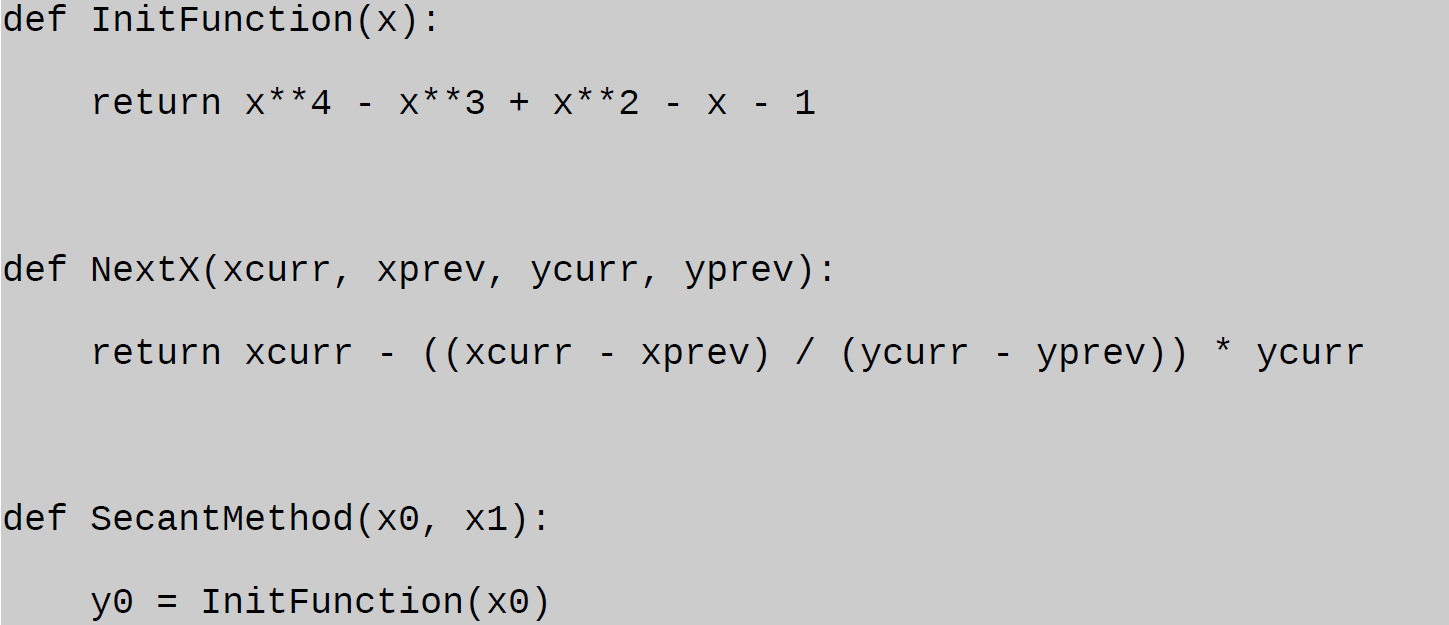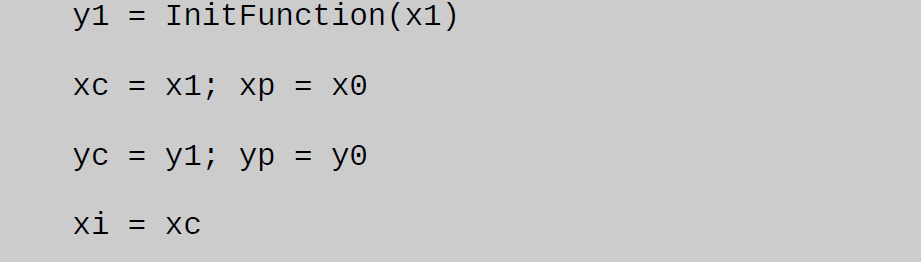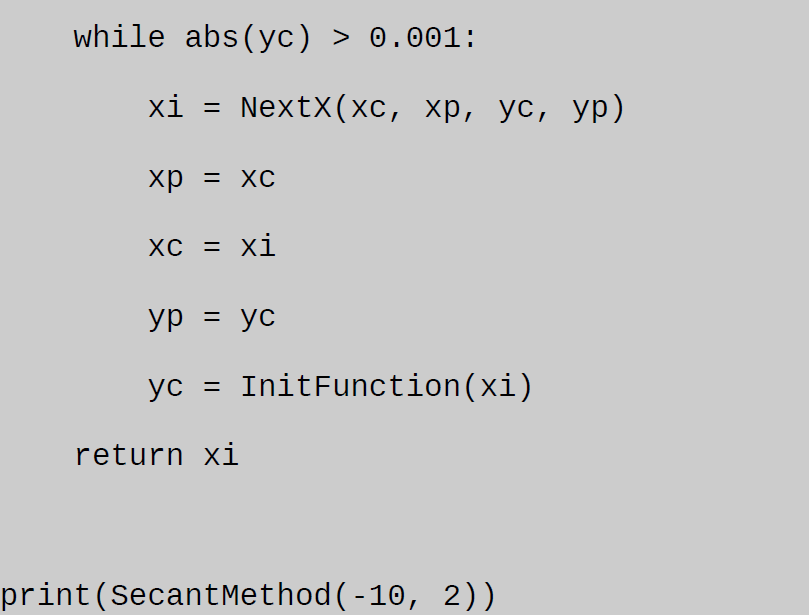Secant method is one of the root-finding algorithms. You can solve equations using this method by hand and with the help of Python code. Here, you can find both secant method examples provided by one of our experts.

Numerical analysis is a complex discipline that requires much time and energy. If you’re too busy with other assignments, we offer you a simple solution. Apply to our experts and they’ll help with assignments. We deal with many disciplines: engineering, architecture, mathematics, IT, and more. Our experts have vast experience in different academic fields. Each of them has to pass specific tests before joining our team.

AssignmentShark guarantees on-time delivery, affordable prices, and 24/7 customer support. We’ll help you with the secant method in Python or any other technical assignment. Place your order and take advantage of all the benefits of our service!